#### You may also like### Euler's Squares

Euler found four whole numbers such that the sum of any two of the numbers is a perfect square...### DOTS Division

Take any pair of two digit numbers x=ab and y=cd where, without loss of generality, ab > cd . Form two 4 digit numbers r=abcd and s=cdab and calculate: {r^2 - s^2} /{x^2 - y^2}.### 2-digit Square

A 2-Digit number is squared. When this 2-digit number is reversed and squared, the difference between the squares is also a square. What is the 2-digit number?

# Hollow Squares

##### Age 14 to 16Challenge Level

Kaitlyn and Zani from Frederick Irwin Anglican School in Australia found how many dots are in each square:
A really easy way to figure out how many soldiers are in the hollow square (or how many dots there are) is to times the outside by itself, for example: if there are 16 dots on the top line you would times 16 by 16 (16$\times$16). Then times the inside of the square by itself, for example: if there are 10 dots on the top line on the inside, you would multiply 10 by itself (10$\times$10).
16$\times$16 = 256
10$\times$10 = 100
Once you know what they equal, you take the 'inside number' from the 'outside number' (256$-$100), which is 156. So now you know that the hollow square has 156 soldiers (or dots).

Mahdi from Mahatma Gandhi International School in India used Alison's method: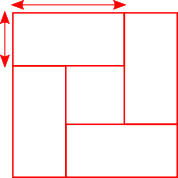$\dfrac{960}4 = 240$

Each rectangle will contain $240$ soldiers because all four rectangles contain the same number of soldiers. Many various dimensions of rectangles for $240$ soldiers can be formed. They are listed below: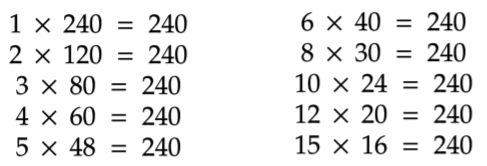Each rectangle with $4$ copies of itself properly arranged can show that it indeed forms a hollow square.

Karrar, Hayder and David from Michaela Community School in the UK, Vid from Srednja Å¡ola ÄŒrnomelj and Siddhant from Singapore International School in India used Charlie's method. Karrar showed how to set up an equation:
We are finding a literal difference of two squares.
$x^2 - y^2 = 960 \Rightarrow (x+y)(x-y) = 960$
$x, y$ are positive integers

This is how Hayder and David then found values for $x$ and $y$ (which they call $a$ and $b$):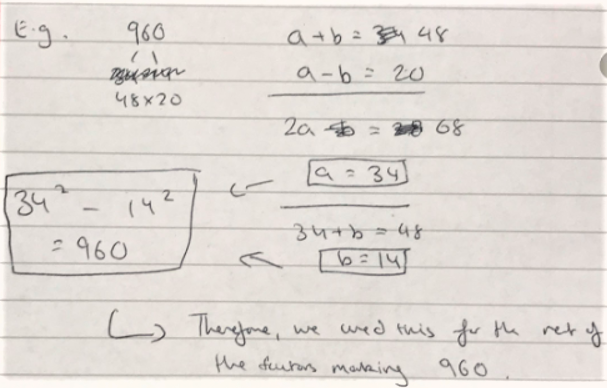Karrar listed all of the factor pairs of 960, but pointed out that not all of them will give symmetric hollow squares. In fact, Hayder and David explained why some of them won't give hollow squares at all: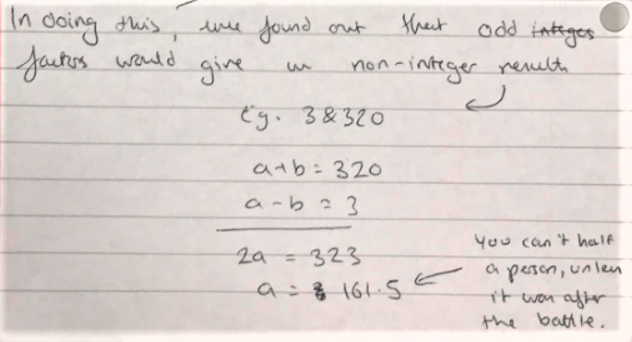Karrar sent in this final answer of all of the symmetrical hollow squares of 196 soldiers: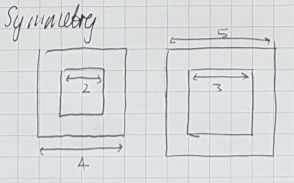We can see that symmetry is created when both numbers are either odd or even

This is because odd $\pm$ odd = even
even $\pm$ even = even

Aim: filter out the results for $x$ and $y$ (from $x^2+y^2=960$) in which $x$ and $y$ are both even or odd.

Here are all 10 of Karrar's answers, which David and Hayder also found. Click here to see a larger version.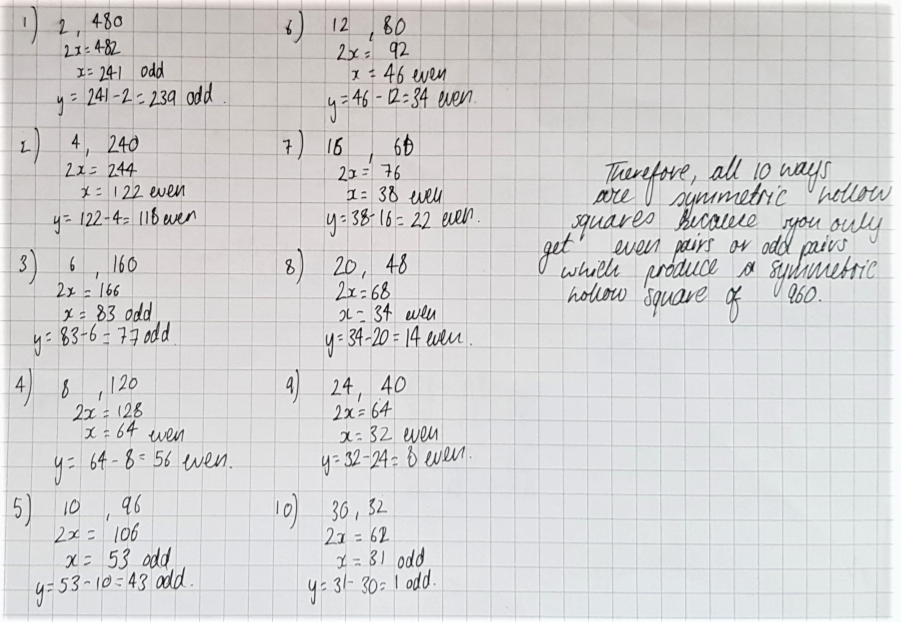Siddhant set up the algebra differently to avoid non-symmetric hollow squares:
We can first take the length of the larger square as $x.$ This means the length of the smaller square is $(x-2c),$ where $c$ is the number of dots between the two squares. It will be $(x-2c)$ because there are $c$ dots separating the squares on both sides.
We can then formulate the expression:

$(x)^2 - (x-2c)^2$

This gives us the total number of soldiers in the hollow square. This uses Charlie's method.

Expanding and simplifying this, we get:

$4c(x-c)$

We can equate this to $960,$ as that is the total number of soldiers said in the question.

$4c(x-c) = 960$
$c(x-c) = 240$

We then find the total number of factor pairs of $240,$ which is exactly $10;$

$1 \times 240, 2 \times 120, 3 \times 80, 4 \times 60, 5 \times 48, 6 \times 40, 8 \times 30, 10 \times 24, 12 \times 20,$
or $15 \times 16$

$c$ is equal to the first number (the smaller number), and $(x-c)$ is equal to the second number (the larger number). Therefore there are ten different ways that the general can arrange the $960$ soldiers in a hollow square.

Notice that this is very similar to Alison's method. In fact, Mahdi and Vid showed how the two methods are related. This is Mahdi's work: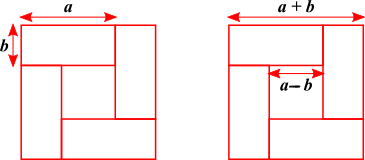\begin{align}(a+b)^2 - (a-b)^2 &= 960\\ \left(a^2 + b^2 + 2ab\right) - \left(a^2+b^2-2ab\right) &= 960\\ a^2 + b^2 + 2ab - a^2 - b^2 - 2ab & = 960\\ 4ab &= 960\end{align}
Vid and Siddhant generalised this to hollow squares with different numbers of soldiers. Vid wrote: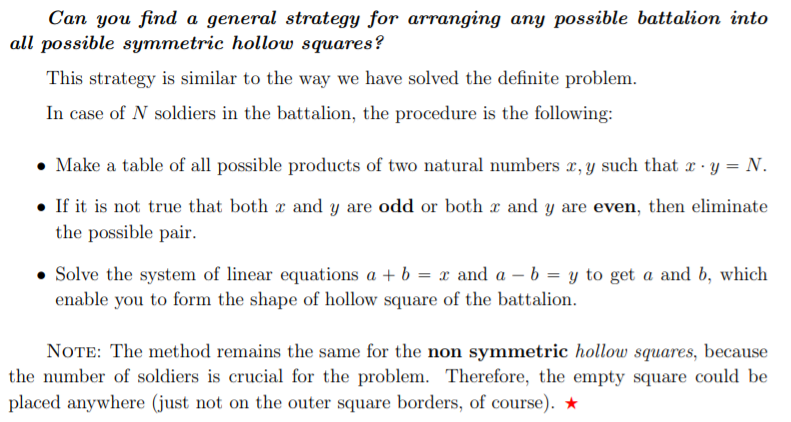Working out which numbers can make symmetric and non-symmetric hollow squares is more complicated. From Siddhant's method (or Alison's method), we can see that hollow squares can only be symmetric if the number of soldiers is a multiple of 4:
$4c(x-c)$ is the number of soldiers in a symmetric hollow square
So the number of soldiers in a symmetric hollow square is always a multiple of $4$

Shyam from Nower Hill and Agathiyan from Hymers College in the UK explained why even numbers which are not multiples of $4$ can never make hollow squares:
Integers with only one 2 able to be factored out of it is not possible to make any hollow square because: when the number is written as $(x+y)(x-y)$, the one 2 must either be a factor of $(x+y)$ or of $(x-y)$, so only one of them is even.
But this does not work as any 2 pairs of numbers when added and subtracted from each other have to create either 2 odd numbers or 2 even numbers, meaning the given circumstance is impossible.

They also found out which odd numbers can make hollow squares:
However, every other number that can't make a symmetrical hollow square (all odd numbers) can make a non-symmetrical square as: $(x+y)(x-y)=x^2-y^2$

For primes, you can make $x-y=1$, so $x+y=\text{the prime}$, so $x$ will be $\frac{prime}{2}+0.5$ and $y$ will be $\frac{prime}2-0.5$. However when $x-y=1$, it will only be a right angle (L shape), not a proper hollow square as for it to be a proper hollow square $x-y\ge2$, $1$ for each border so no primes can make a symmetrical hollow square.

Meanwhile other odd numbers can work if $x-y$ is one of their factors and $x+y$ is the other factor in the factor pair.
(in this case, $x+y$ and $x-y$ will both be odd)